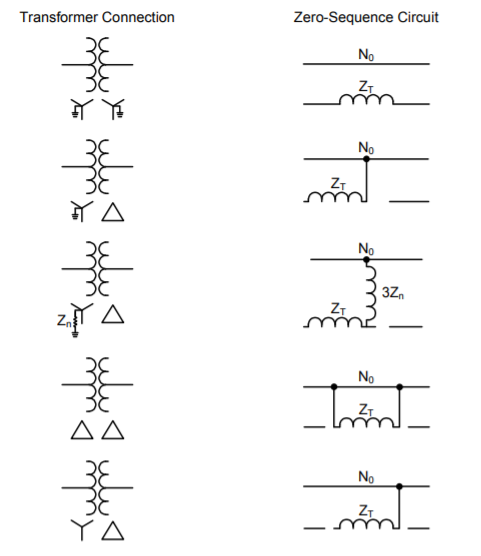Like any other elements of power system, transformer can also be represented by its corresponding sequence network. However, the characteristic of transformer is unique which is dependent on how it is banked. The transformer representation in its zero sequence network can be more complex compare with its positive and negative components.

Below are the sequence network of a three phase transformer based on it's banking connection.

1. Y-Y Transformer with grounding

When a transformer has at least two grounded-wye windings, zero-sequence current can be transformed between the grounded-wye windings. The I0 currents will add up to 3I0 in the neutral and return through ground or the neutral conductor. The I0 currents will be transformed into the secondary windings and flow in the secondary circuit. Any impedance between the transformer neutral points and ground must be represented in the zero-sequence network as three times its value to correctly account for the zero-sequence voltage drop across it. Below on the left is a three-phase diagram of a grounded-wye, grounded-wye transformer connection with its zero-sequence network model on the right. Notice the resistance in the neutral of the secondary winding is modeled by 3R in the zero-sequence network model.Zero-Sequence model of Y-Y transformer (both grounded)

2. Delta-Wye (wye is grounded)

When a transformer has a grounded-wye winding and a delta winding, zerosequence currents will be able to flow through the grounded-wye winding of the transformer. The zero-sequence currents will be transformed into the delta winding where they will circulate in the delta without leaving the terminals of the transformer. Because the zero-sequence current in each phase of the delta winding is equal and in phase, current does not need to enter or exit the delta winding. Below on the left is a three-phase diagram of a grounded-wye-delta transformer connection with its zero-sequence network model on the right.Zero Sequence model of Delta-Wye (grounded) transformer

As we can see, all WYE connections are grounded. In case, it is solidly grounded and no impedance exist in the ground conductor then simply eliminate it and replace it with short circuit (zero impedance).

All other models can be represented by the figure below,General Representation of Transformer Zero-Sequence Network

As shown from the diagram above, there is no zero sequence current that will flow to the reference bus of faulted system for delta connection and Wye (ungrounded).

This diagram can be explained by the rules applied on the zero-sequence networks of transformer.
1. When magnetizing current is neglected, transformer primary would carry current only if there is current flow on the secondary side.
2. Zero sequence currents can flow in the legs of wye connection only if it is grounded and provide effective path to the ground.
3. No zero sequence currents can flow in the lines of delta connection as no return path is avaiable for these currents.